Example Questions

← Previous 1 3 4

Example Question #1 : How To Simplify An Expression

What is the value of x when 2x - 7 =  -5x + 28

-5

5

7

-7

5

Explanation:

We need to solve for x. To do so, first move the x values to the left of the = sign and all other values to the right. 2x + 5x = 28 + 7.  Then add like terms: 7x = 35. In order to get x all by itself, we then divide both sides by 7. X = 5.

Example Question #1 : How To Simplify An Expression

If 4x – 6 = 18, what is 3x3 – 4x + 1?

300

97

625

-1

625

Explanation:

First we have to solve for x using the first equation:

4x-6 = 18

4x = 24

x = 6

Next, we have to plug in our value for x into the second equation:

3(6)3 – 4(6) + 1

3 (216) – 24 +1 = 625

Example Question #1 : Simplifying Expressions

What is the value of z when 2z + 3 = 3z – 5?

4

1/8

-8

8

8

Explanation:

Solve one equation with one variable by moving the constants to one side of the equal sign and the variables to the other.  2z + 3 = 3z – 5 is solved by adding 5 -2z to both side of the equation and ending up with z = 8

Example Question #1 : How To Simplify An Expression

Given that 3x + 7 = 4x – 2, what is the value of x ?

1

5/7

9

9/7

9

Explanation:

First, we must solve the equation for x by subtracting 3x from both sides:

3x – 3x + 7 = 4x – 3x – 2

7 = x – 2

Then we must add 2 to both sides:

7 + 2 = x – 2 + 2

9 = x

Example Question #2 : Simplifying Expressions

If a = 4 and b =3 then:  2a2 + 3ab – 7  is?

61

73

37

– 2

61

Explanation:

Substitute the values of a and b into the equation. Then

2a2 + 3ab – 7

(2 x 42 ) + (3 x 4 x 3) – 7

(2 x 16) + (36) -7

32 + 36 – 7

61

Example Question #1 : How To Simplify An Expression

Which of the following expressions are equivalent to b for all non-zero real numbers a, b, x, and y such that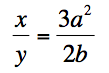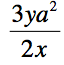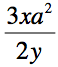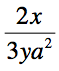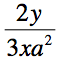None of the answers are correctExplanation:

Cross multiply to get 2xb = 3ya2, then divide by 2x to get b on one side of the equal sign by itself.

Example Question #6 : How To Simplify An Expression

A store in California specializes in the sale of custom t-shirts and is growing rapidly. In their second year of business, they doubled the sales from the first year, and in the third year, they sold 50,000 more t -shirts than their second year. In their fourth year, they doubled their sales from the third year. If in the fourth year of business, the store sold 300,000 shirts, how many did they sell in the second year?

150,000

25,000

50,000

250,000

100,000

100,000

Explanation:

This question is a bit wordy, so it is tough to not get lost in it, it usually helps to write down the pertinent information on a separate sheet of paper. In this case, you should have written that year 4 = 2 x year 3 = 300,000

so, 300,000 = 2 x year 3

divide each side by 2 and we get

150,000 sold in year 3. We are told that year 3 = year 2 +50,000

so, subtracting 50,000 from each side yields

year 3 - 50,000 = total sales from year 2

150,000 - 50,000 = total sales for year 2 = 100,000

Example Question #1 : How To Simplify An Expression

Which of the following values is the greatest?

28

49

164

324

82

324

Explanation:

In order to compare each quantity, we need to rewrite each one using the same base. A common base that would be easy to use would be 2, because we can write 2, 4, 8, 16, and 32 all as a power of 2. We also need to remember that when taking an exponent to an exponent, we have to multiple the two exponents together.

28 = 28

82 = (23)2 = 26

164 = (24)4 = 216

49 = (22)9 = 218

324 = (25)4 = 220

When we compare all of the numbers as powers of 2, we realize that 220 is the largest. Thus, the answer is 324.

Example Question #1 : Simplifying Expressions

Which of the following expressions is equivalent to 4x2 + 10x – 6?

2(2x +1)(x – 3)
(2x +1)(2x – 6)
2(2x – 1)(x + 3)
(2x – 2)(2x + 3)
(4x + 2)(x – 3)
Correct answer: 2(2x – 1)(x + 3)
Explanation:

First, pull out a common factor of 2 to get 2(2x2 + 5x – 3). Then factor the quadratic so that the x terms add to 5x and the numbers multiply to - 3, resulting in 2(2x – 1)(x + 3).

Example Question #9 : How To Simplify An Expression

What is the value of x when 3x + 5 = 2x – 7?

–9

5/12

12/5

12

–12